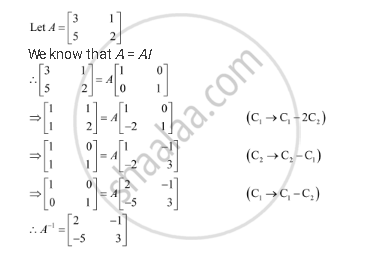Share

# Find the Inverse of Each of the Matrices, If It Exists [(3,1),(5,2)] - CBSE (Science) Class 12 - Mathematics

#### Question

Find the inverse of each of the matrices, if it exists.

[(3,1),(5,2)]

#### SolutionIs there an error in this question or solution?

#### Video TutorialsVIEW ALL 

Solution Find the Inverse of Each of the Matrices, If It Exists [(3,1),(5,2)] Concept: Invertible Matrices.
S